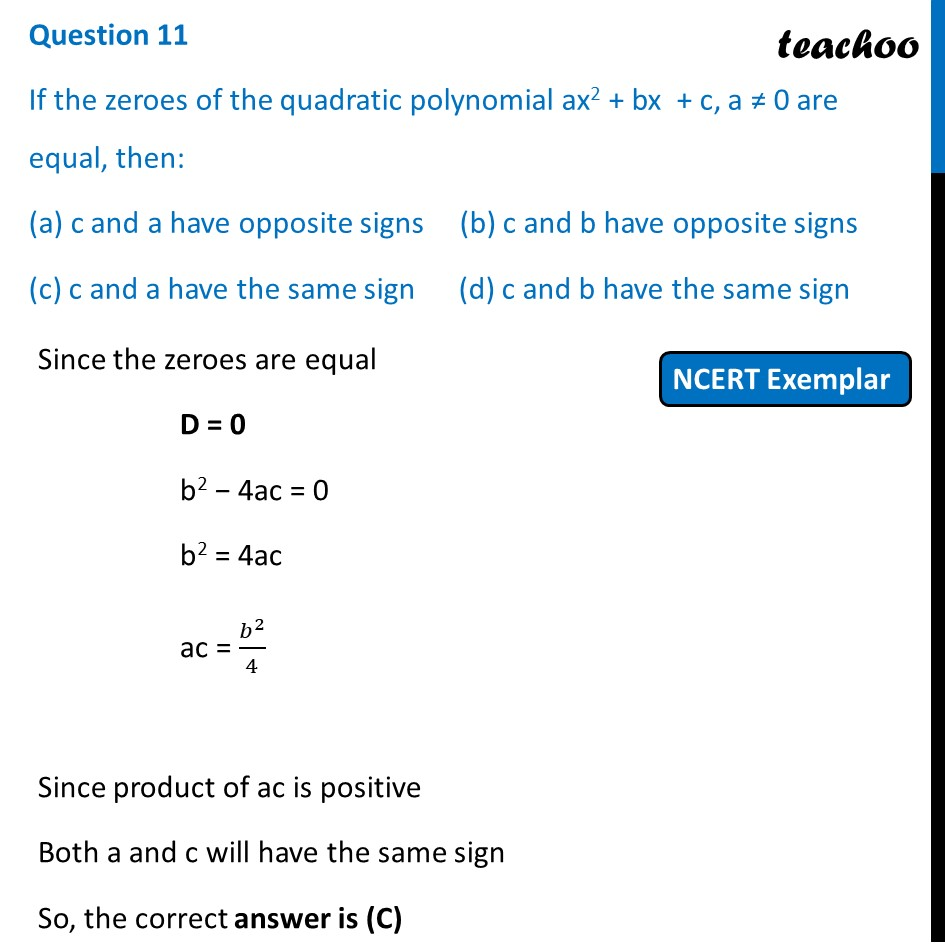## (c) c and a have the same sign           (d) c and b have the same sign1. Chapter 2 Class 10 Polynomials (Term 1)
2. Serial order wise
3. MCQs from NCERT Exemplar

Transcript

Question 11 If the zeroes of the quadratic polynomial ax2 + bx + c, a ≠ 0 are equal, then: (a) c and a have opposite signs (b) c and b have opposite signs (c) c and a have the same sign (d) c and b have the same sign Since the zeroes are equal D = 0 b2 − 4ac = 0 b2 = 4ac ac = 𝑏^2/4 Since product of ac is positive Both a and c will have the same sign So, the correct answer is (C)

MCQs from NCERT Exemplar